# 小哥哥小姐姐，来尝尝Async函数这块语法糖

ES7（ECMAScript 2016）推出了Async函数（`async/await`），实现了以顺序、同步代码的编写方式来控制异步流程，彻底解决了困扰JavaScript开发者的“回调地狱”问题。比如，之前需要嵌套回调的异步逻辑：

``````const result = [];
// pseudo-code, ajax stand for an asynchronous request
ajax('url1', function(err, data){
if(err) {...}
result.push(data)
ajax('url2', function(err, data){
if(err) {...}
result.push(data)
})
})
console.log(result)``````

``````async function example() {
const r1 = await new Promise(resolve =>
setTimeout(resolve, 500, 'slowest')
)
const r2 = await new Promise(resolve =>
setTimeout(resolve, 200, 'slow')
)
return [r1, r2]
}

example().then(result => console.log(result))
// ['slowest', 'slow']``````

Async函数需要在`function`前面添加`async`关键字，同时内部以`await`关键字来“阻塞”异步操作，直到异步操作返回结果，然后再继续执行。在没有Async函数以前，我们无法想象下面的异步代码可以直接拿到结果：

``````const r1 = ajax('url')
console.log(r1)
// undefined``````

## 1. 当前JavaScript编程主要是异步编程

Web应用或开发重交互的特征越来越明显，意味着什么？意味着按照浏览器这个运行时的特性，页面在首次加载过程中，与JavaScript相关的主要任务就是加载基础运行库和扩展库（包括给低版本浏览器打补丁的脚本），然后初始化和设置页面的状态。首次加载之后，用户对页面的操作、数据I/O以及DOM更新，就全部交由异步JavaScript脚本管理。所以，目前JavaScript编程最大的应用是Web交互，而Web交互的核心就是异步逻辑。

``````var req = new XMLHttpRequest();
req.open('GET', url);

if (req.status == 200) {
processData(req.response);
}
};

req.onerror = function () {
console.log('Network Error');
};

req.send(); ``````

``````// Node.js
if (error) {
// ...
}
console.log(data);
}
);``````

## 2. Promise

Promise，人们普遍的理解就是：“Promise是一个未来值的占位符”。也就是说，从语义上讲，一个Promise对象代表一个对未来值的“承诺”（promise），这个承诺将来如果“兑现”（fulfill），就会“解决”（resolve）为一个有意义的数据；如果“拒绝”（reject），就会“解决”为一个“拒绝理由”（rejection reason），就是一个错误消息。

Promise对象的状态很简单，一生下来的状态是`pending`（待定），将来兑现了，状态变成`fulfilled`；拒绝了，状态变成`rejected``fulfilled``rejected`显然是一种“确定”（settled）状态。以上状态转换是不可逆的，所以Promise很单纯，好控制，哈哈。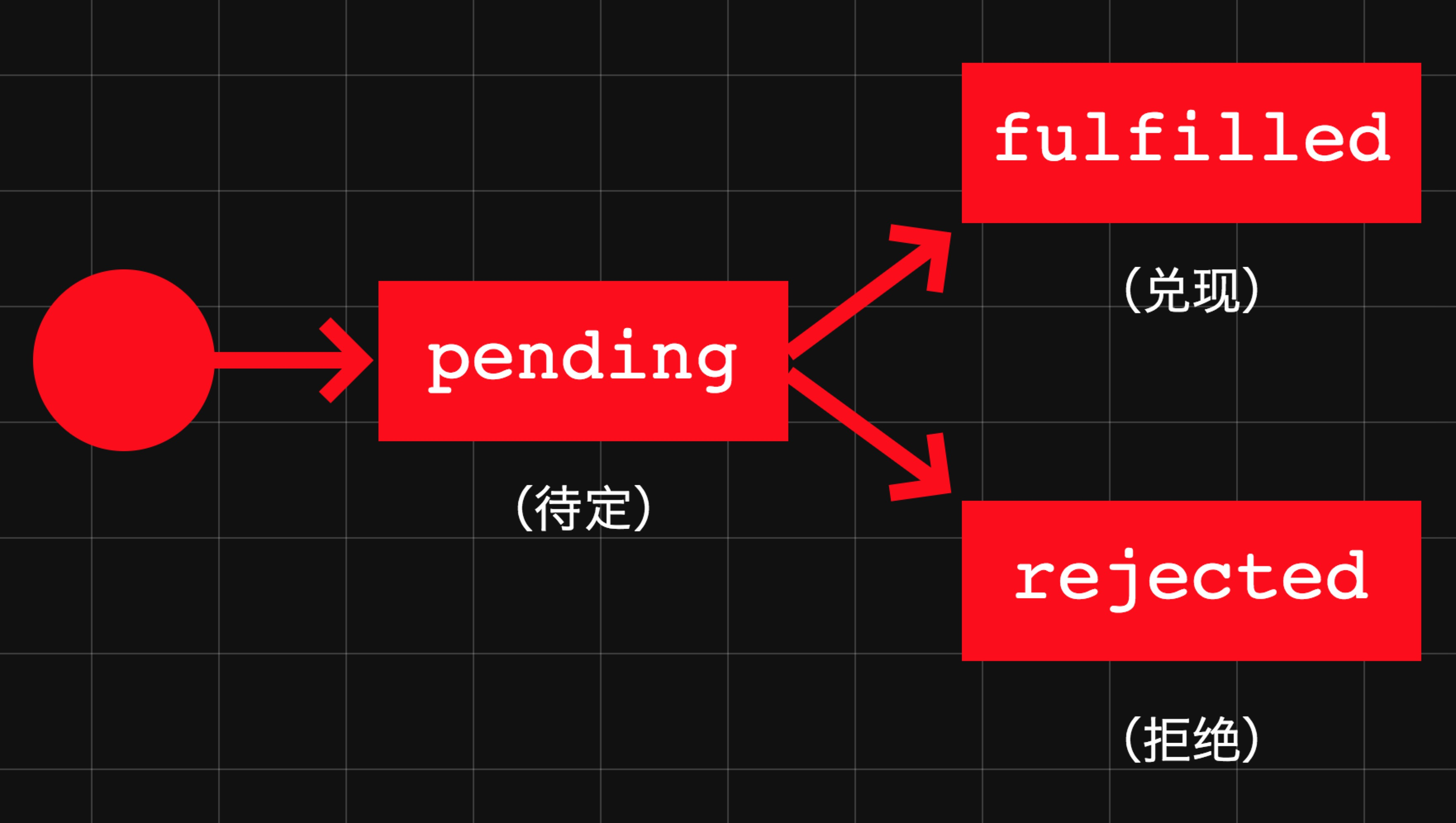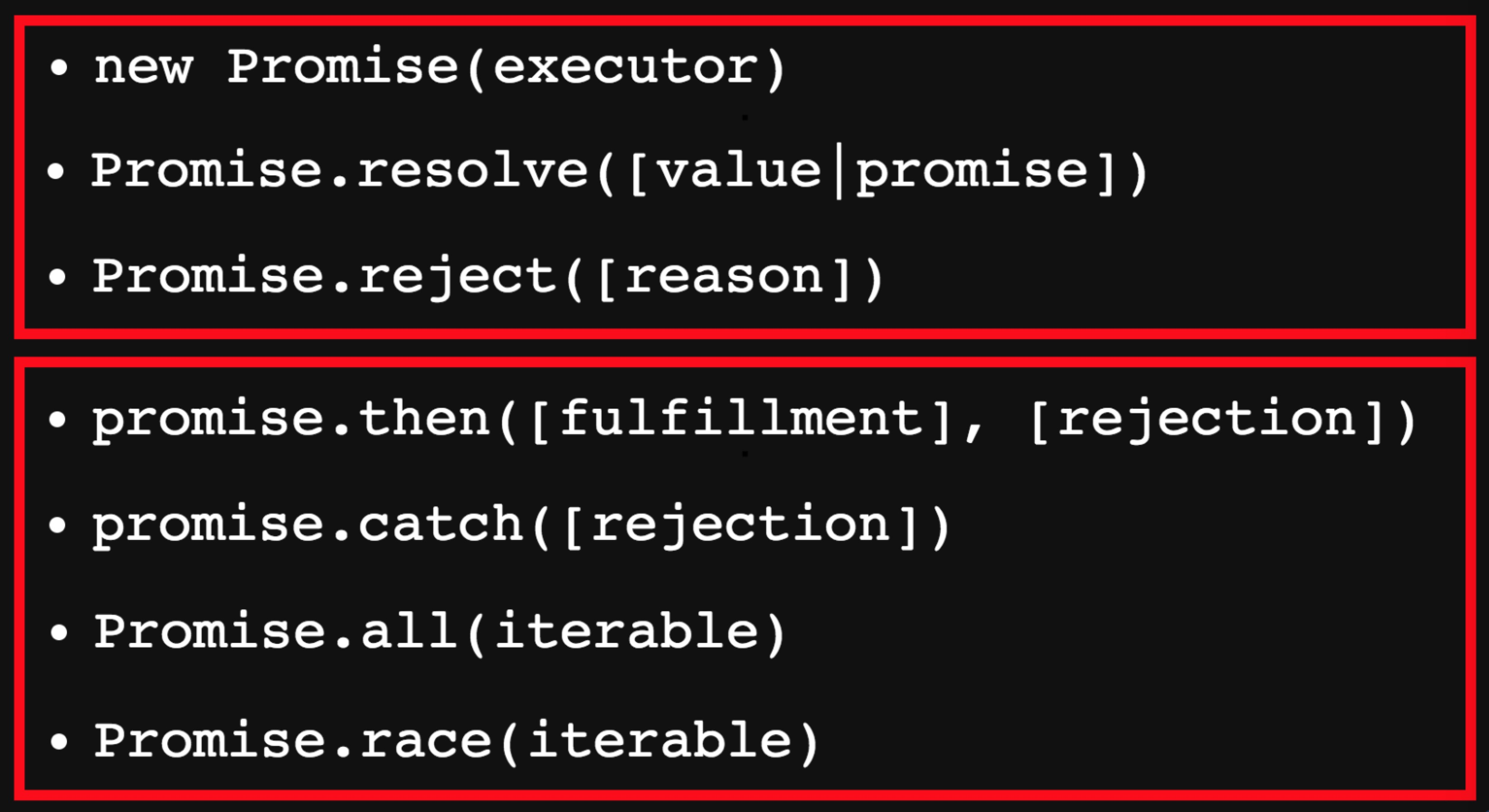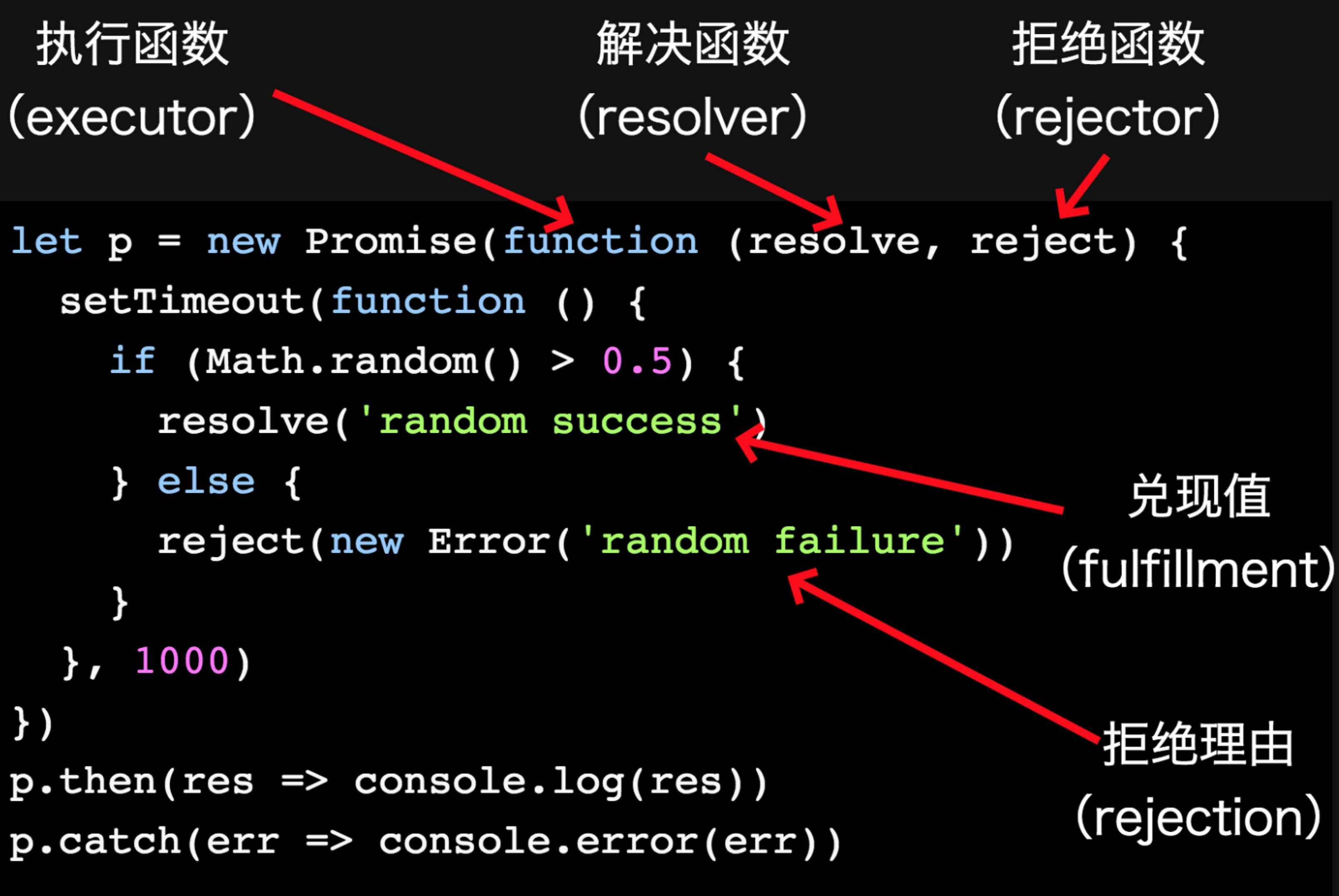``````p.then(res => { // 兑现反应函数
// res === 'random success'
})
p.catch(err => { // 拒绝反应函数
// err === 'random failure'
})``````

``````// 通过一个.then()方法同时注册兑现和拒绝函数
p.then(
res => {
// handle response
},
err => {
// handle error
}
)
// 通过.then()方法只注册一个函数：兑现函数
p.then(res => {
// handle response
})
// 通过.then()方法只传入拒绝函数，兑现函数的位置传null
p.then(null, err => {
// handle error
})``````

## 3. Iterator

``````interface IteratorResult {
done: boolean;
value: any;
}
interface Iterator {
next(): IteratorResult;
}
interface Iterable {
[Symbol.iterator](): Iterator
}``````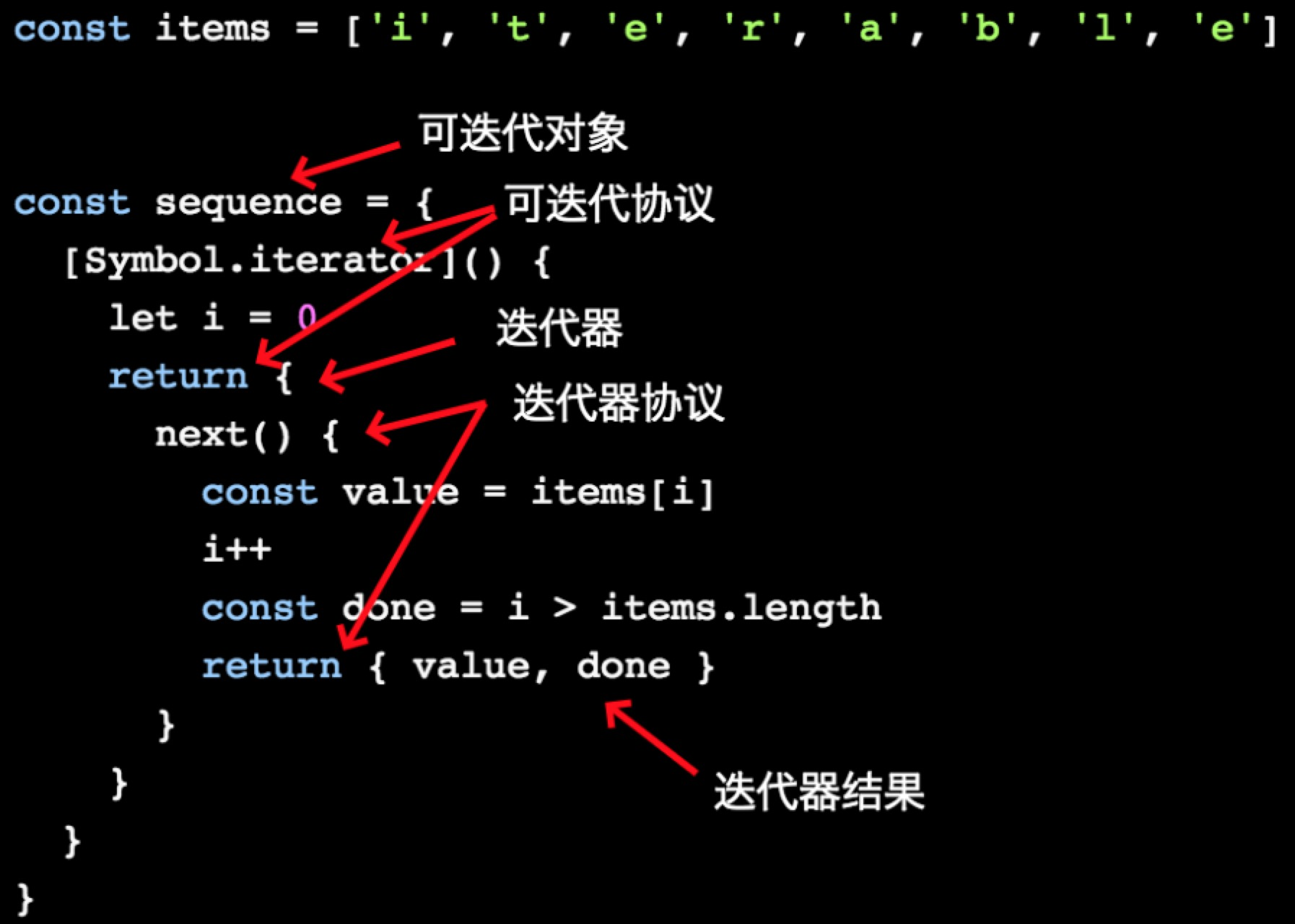• `for...of`
• `[...iterable]`
• `Array.from(iterable)`

• `for...in`
• `Array#forEach`

``````for (const item of sequence) {
console.log(item)
// 'i'
// 't'
// 'e'
// 'r'
// 'a'
// 'b'
// 'l'
// 'e'
}

console.log([...sequence])
// ['i', 't', 'e', 'r', 'a', 'b', 'l', 'e']

console.log(Array.from(sequence))
// ['i', 't', 'e', 'r', 'a', 'b', 'l', 'e']``````

``````const random = {
[Symbol.iterator]: () => ({
next: () => ({ value: Math.random() })
})
}

// 运行这行代码会怎么样？
[...random]
// 这行呢？
Array.from(random)``````

``````interface IteratorResult {
done: boolean;
value: any;
}``````

``````interface Iterator {
next(): IteratorResult;
}``````

``````interface Iterable {
[Symbol.iterator](): Iterator
}``````

``````// 运行这行代码会怎么样？
[...random]
// 这行呢？
Array.from(random)``````

``````const [one, another] = random  // 解析赋值，取得前两个随机数
console.log(one)
// 0.23235511826351285
console.log(another)
// 0.28749457537196577

for (const value of random) {
if (value > 0.8) { // 退出条件，随机数大于0.8则中断循环
break
}
console.log(value)
}``````

## 4. Generator

``````interface Generator extends Iterator {
next(value?: any): IteratorResult;
[Symbol.iterator](): Iterator;
throw(exception: any);
}``````

``````function *gen() {
yield 'a'
yield 'b'
return 'c'
}``````

``````const chars = gen()
typeof chars[Symbol.iterator] === 'function' // chars是可迭代对象
typeof chars.next === 'function'  // chars是迭代器
chars[Symbol.iterator]() === chars  // chars的迭代器就是它本身
console.log(Array.from(chars))  // 可以对它使用Array.from
// ['a', 'b']
console.log([...chars]) // 可以对它使用Array.from
// ['a', 'b']``````

``````function *gen(x) {
const y = x * (yield)
return y
}

const it = gen(6)
it.next()
// {value: undefined, done: false}
it.next(7)
// {value: 42, done: true}``````

1. 调用`gen(6)`创建生成器的迭代器`it`（前面说了，生成器包含迭代器及返回它的生成器函数），传入数值6。
2. 调用`it.next()`启动生成器。此时生成器函数的代码执行到第一个`yield`表达式处暂停，并返回`undefined`。（`yield`并没闲着，它看后面没有显式要返回的值，就只能返回默认的`undefined`。）
3. 调用`it.next(7)`恢复生成器执行。此时`yield`接收到传入的数值7，立即恢复生成器函数代码的执行，并把自己替换成数值7。
4. 代码计算：`6 * 7`，得到42，并把42赋给变量`y`，最后返回`y`
5. 生成器函数最终返回的值就是：`{value: 42, done: true}`

1. `yield`表达式返回序列中下一个值
2. `return`语句返回生成器函数的值（`{ done: true }`
3. `throw`语句完全停止生成器执行（后面会详细解释）
4. 到达生成器函数最后，隐式返回`{ value: undefined, done: true}`

### 4.1 同步错误处理

``````function *main() {
const x = yield "Hello World";
yield x.toLowerCase(); // 导致异常！
}

const it = main();
it.next().value; // Hello World
try {
it.next( 42 );
} catch (err) {
console.error(err); // TypeError
}``````

``````function *main() {
var x = yield "Hello World";
console.log('never gets here');
}

const it = main();
it.next().value; // Hello World
try {
it.throw('Oops'); // `*main()`会处理吗？
} catch (err) {   // 没有！
console.error(err); // Oops
}``````

### 4.2 异步迭代生成器

``````const r1 = ajax('url')
console.log(r1)
// undefined``````

``````const r1 = yield ajax('url')
console.log(r1)
// 这次r1就是真正的响应结果了``````

``````function foo(x,y) {
return request(
"http://some.url.1/?x=" + x + "&y=" + y
);
}

foo(11, 31)
.then(
function(text){
console.log(text);
},
function(err){
console.error(err);
}
);``````

``````function foo(x, y) {
return request(
"http://some.url.1/?x=" + x + "&y=" + y
);
}
function *main() {
try {
const result = yield foo(11, 31);  // 异步函数调用！
console.log( result );
} catch (err) {
console.error( err );
}
}
const it = main();
const p = it.next().value; // 启动生成器并取得Promise `p`

p.then( // 等待Promise `p`解决
function(res){
it.next(res);  // 把`text`传给`*main()`并恢复其执行
},
function(err){
it.throw(err);  // 把`err`抛到`*main()`
}
);``````

（当然，如果异步请求发生错误，在`p`的拒绝反应函数中也会通过`it.throw(err)`把错误抛给生成器函数。但这个现在不重要。）

## 5. 通用的生成器运行程序

``````function example() {
return run(function *() {
const r1 = yield new Promise(resolve =>
setTimeout(resolve, 500, 'slowest')
)
const r2 = yield new Promise(resolve =>
setTimeout(resolve, 200, 'slow')
)
return [r1, r2]
})
}

example().then(result => console.log(result))
// ['slowest', 'slow']``````

``````function run(gen) {
const it = gen();
return Promise.resolve()
.then( function handleNext(value){
let next = it.next( value );
return (function handleResult(next){
if (next.done) {
return next.value;
} else {
return Promise.resolve( next.value )
.then(
handleNext,
function handleErr(err) {
return Promise.resolve(
it.throw( err )
)
.then( handleResult );
}
);
} // if...else
})(next); // handleResult(next)
}); // handleNext(value)
}``````

### 供参考的“人肉”执行过程

（调用`run`的代码见本节开头。）

`handleResult(next)`内部，首先检查`next.done`，不等于`true`，进入`else`子句。此时通过`Promise.resolve(next.value)`包装`next.value`：等待返回的Promise解决，解决之后拿到字符串值`'Slowest'`，然后传给兑现反应函数`handleNext(value)`

``````function run(generator) {
return new Promise((resolve, reject) => {
const it = generator()
step(() => it.next())
function step(nextFn) {
const result = runNext(nextFn)
if (result.done) {
resolve(result.value)
return
}
Promise
.resolve(result.value)
.then(
value => step(() => it.next(value)),
err => step(() => it.throw(err))
)
}
function runNext(nextFn) {
try {
return nextFn()
} catch (err) {
reject(err)
}
}
})
}``````

## 6. 为什么说Async函数是语法糖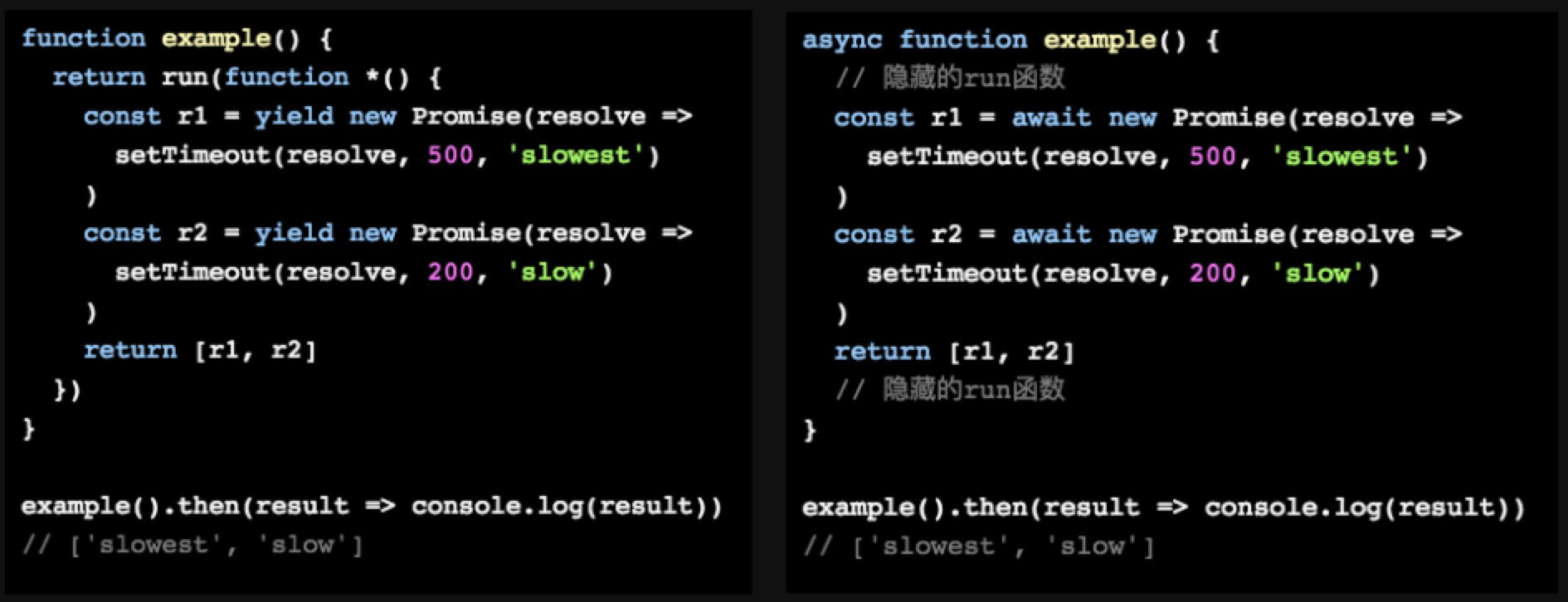``````async function example() {
const r1 = await new Promise(resolve =>
setTimeout(resolve, 500, 'slowest')
)
const r2 = await new Promise(resolve =>
setTimeout(resolve, 200, 'slow')
)
return [r1, r2]
}

example().then(result => console.log(result))
// ['slowest', 'slow']``````

## 7. 参考资料

• ECMAScript 2018
• Practical Modern JavaScript
• You Don't Know JS: Async & Performance
• Understanding ECMAScript 6
• Exploring ES6